# ggpie - A ggplot2 extension to create pie, donut and rose pie plot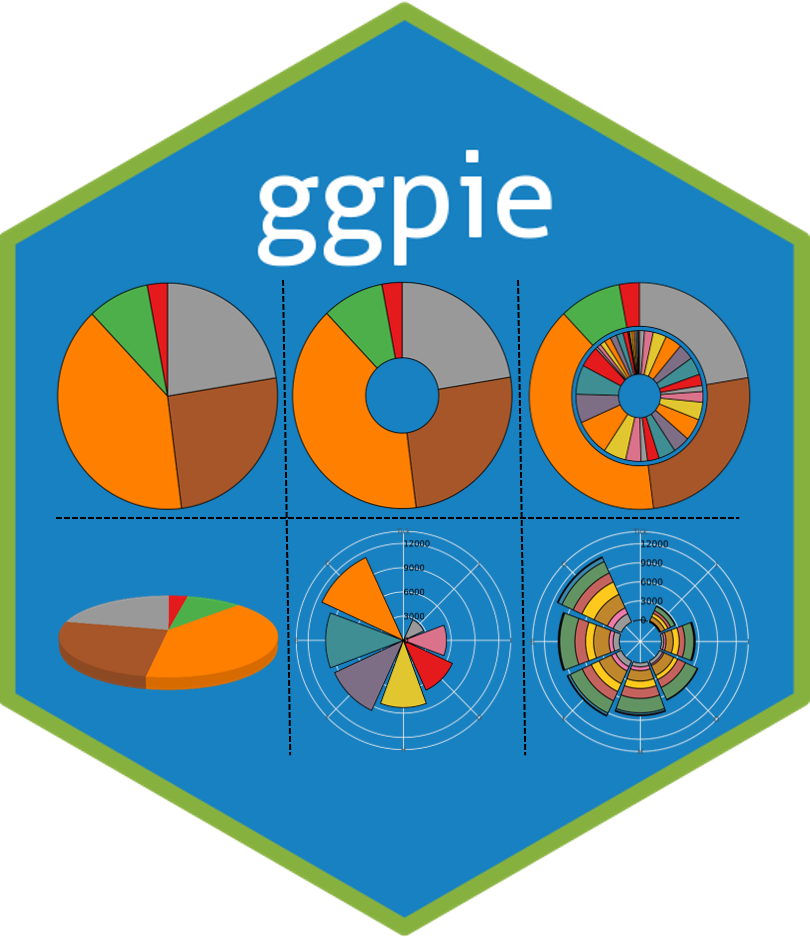## Introduction

`ggpie` aims to create pie (2D and 3D), donut and rose pie plot with the `ggplot2` plotting system which implemented the grammar of graphics. It contains five main functions:

• `ggpie`: Create 2D pie plot with single group variable.
• `ggdonut`: Create 2D donut plot with single group variable.
• `ggnestedpie`: Create 2D nested pie plot with two group variables.
• `ggpie3D`: Create 3D pie plot with single group variable.
• `ggrosepie`: Create rose pie plot with single or two group variables.

## Installation

You can install the released version of `ggpie` from CRAN with:

``install.packages("ggpie")``

Or install the package via the Github repository:

``````# install.package("remotes")   #In case you have not installed it.
remotes::install_github("showteeth/ggpie")``````

## Citation

``````citation("ggpie")
#>
#> To cite ggpie in publications use:
#>
#>   Yabing Song (2022). ggpie: Create Pie, Donut and Rose Pie Plot with
#>   'ggplot2'. R package version 0.2.2.
#>   https://CRAN.R-project.org/package=ggpie
#>
#> A BibTeX entry for LaTeX users is
#>
#>   @Manual{,
#>     title = {ggpie: Create Pie, Donut and Rose Pie Plot with 'ggplot2'.},
#>     author = {Yabing Song},
#>     note = {R package version 0.2.2},
#>     year = {2022},
#>     url = {https://CRAN.R-project.org/package=ggpie},
#>   }``````

## Usage

### Preapre data

``````library(ggpie)
library(ggplot2)
data(diamonds)
# check data used
str(diamonds)
#> tibble[,10] [53,940 × 10] (S3: tbl_df/tbl/data.frame)
#>  \$ carat  : num [1:53940] 0.23 0.21 0.23 0.29 0.31 0.24 0.24 0.26 0.22 0.23 ...
#>  \$ cut    : Ord.factor w/ 5 levels "Fair"<"Good"<..: 5 4 2 4 2 3 3 3 1 3 ...
#>  \$ color  : Ord.factor w/ 7 levels "D"<"E"<"F"<"G"<..: 2 2 2 6 7 7 6 5 2 5 ...
#>  \$ clarity: Ord.factor w/ 8 levels "I1"<"SI2"<"SI1"<..: 2 3 5 4 2 6 7 3 4 5 ...
#>  \$ depth  : num [1:53940] 61.5 59.8 56.9 62.4 63.3 62.8 62.3 61.9 65.1 59.4 ...
#>  \$ table  : num [1:53940] 55 61 65 58 58 57 57 55 61 61 ...
#>  \$ price  : int [1:53940] 326 326 327 334 335 336 336 337 337 338 ...
#>  \$ x      : num [1:53940] 3.95 3.89 4.05 4.2 4.34 3.94 3.95 4.07 3.87 4 ...
#>  \$ y      : num [1:53940] 3.98 3.84 4.07 4.23 4.35 3.96 3.98 4.11 3.78 4.05 ...
#>  \$ z      : num [1:53940] 2.43 2.31 2.31 2.63 2.75 2.48 2.47 2.53 2.49 2.39 ...``````

### Pie plot

#### no label

Pie plot with no label:

``````# with no label
ggpie(data = diamonds, group_key = "cut", count_type = "full",label_type = "none")``````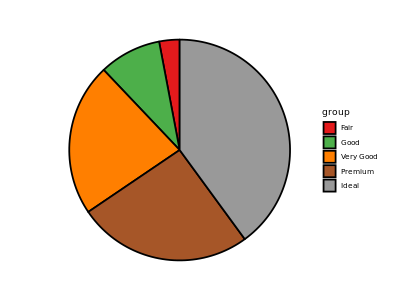#### circle label

circle label and out of pie:

``````ggpie(data = diamonds, group_key = "cut", count_type = "full",
label_info = "all", label_type = "circle",
label_size = 4, label_pos = "out")``````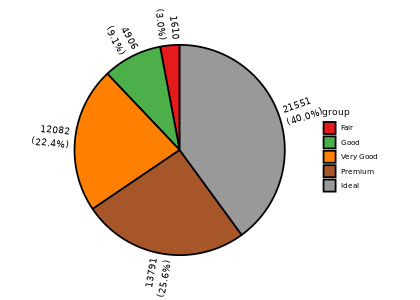circle label and in pie plot, with no split:

``````ggpie(data = diamonds, group_key = "cut", count_type = "full",
label_info = "all", label_type = "circle", label_split = NULL,
label_size = 4, label_pos = "in")``````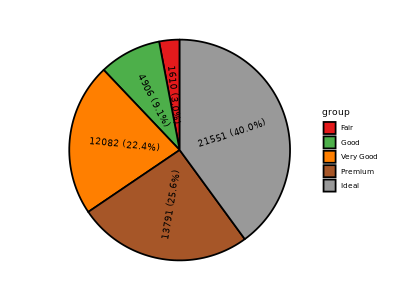#### horizon label

horizon label and in pie plot, with no split:

``````ggpie(data = diamonds, group_key = "cut", count_type = "full",
label_info = "all", label_type = "horizon", label_split = NULL,
label_size = 4, label_pos = "in")``````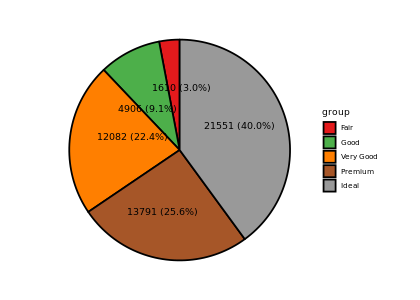horizon label and in pie plot, split with space:

``````ggpie(data = diamonds, group_key = "cut", count_type = "full",
label_info = "all", label_type = "horizon",
label_size = 4, label_pos = "in")``````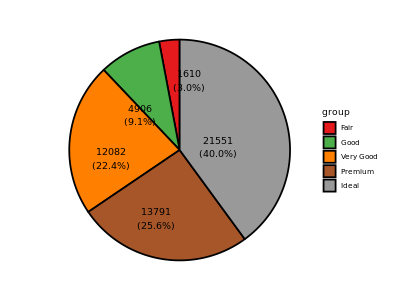horizon label and out pie plot, with no split:

``````ggpie(data = diamonds, group_key = "cut", count_type = "full",
label_info = "all", label_type = "horizon",
label_size = 4, label_pos = "out" )``````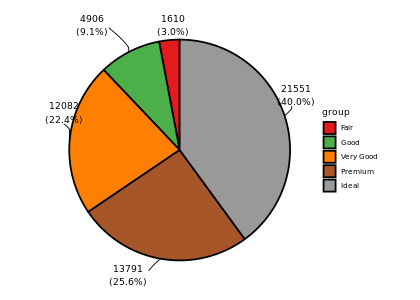With `label_threshold`, `ggpie` will move label below `label_threshold` to out of pie. In this example, all labels below 10% are moved to out of pie:

``````ggpie(data = diamonds, group_key = "cut", count_type = "full",
label_info = "all", label_type = "horizon", label_split = NULL,
label_size = 4, label_pos = "in", label_threshold = 10)``````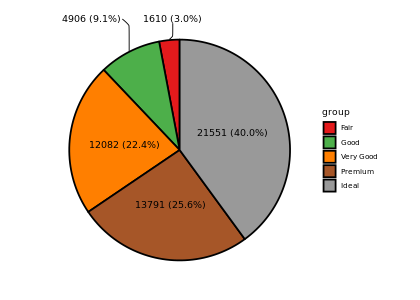### Donut plot

#### no label

``````# with no label
ggdonut(data = diamonds, group_key = "cut", count_type = "full",label_type = "none")``````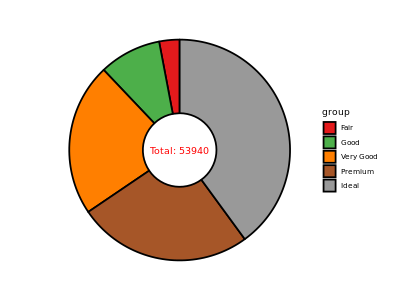#### circle label

circle label and out of pie:

``````ggdonut(data = diamonds, group_key = "cut", count_type = "full",
label_info = "all", label_type = "circle",
label_size = 4, label_pos = "out")``````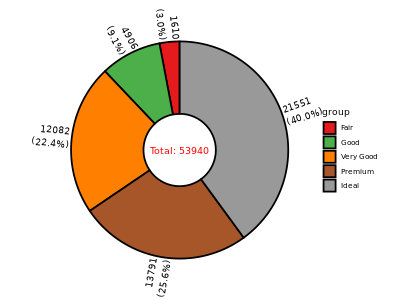circle label and in pie plot, with no split:

``````ggdonut(data = diamonds, group_key = "cut", count_type = "full",
label_info = "all", label_type = "circle", label_split = NULL,
label_size = 4, label_pos = "in")``````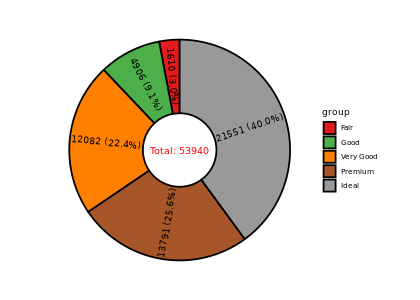#### horizon label

horizon label and in pie plot, with no split:

``````ggdonut(data = diamonds, group_key = "cut", count_type = "full",
label_info = "all", label_type = "horizon", label_split = NULL,
label_size = 4, label_pos = "in")``````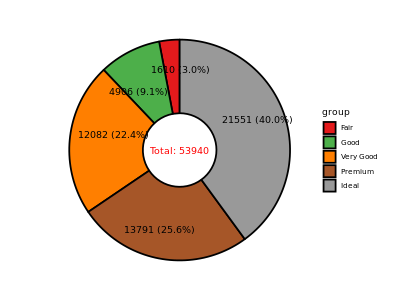horizon label and in pie plot:

``````ggdonut(data = diamonds, group_key = "cut", count_type = "full",
label_info = "all", label_type = "horizon",
label_size = 4, label_pos = "in")``````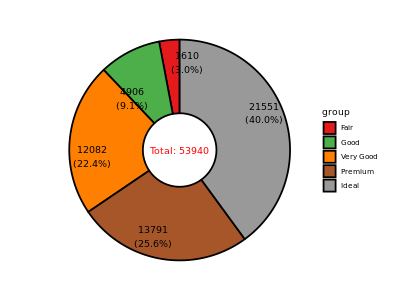horizon label and out of pie plot, with no split:

``````ggdonut(data = diamonds, group_key = "cut", count_type = "full",
label_info = "all", label_type = "horizon", label_split = NULL,
label_size = 4, label_pos = "out")``````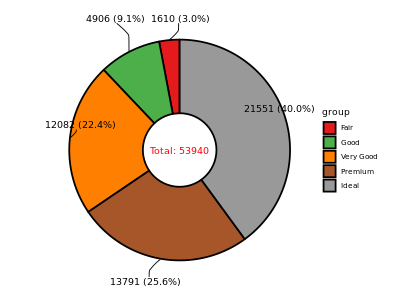horizon label and out of pie plot:

``````ggdonut(data = diamonds, group_key = "cut", count_type = "full",
label_info = "all", label_type = "horizon",
label_size = 4, label_pos = "out")``````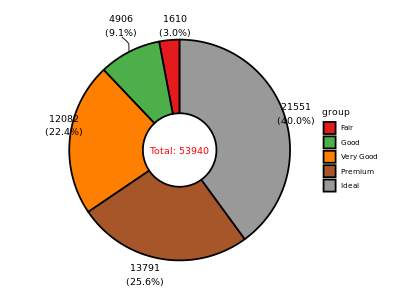With `label_threshold`, `ggpie` will move label below `label_threshold` to out of donut. In this example, all labels below 10% are moved to out of donut:

``````ggdonut(data = diamonds, group_key = "cut", count_type = "full",
label_info = "all", label_type = "horizon", label_split = NULL,
label_size = 4, label_pos = "in", label_threshold = 10)``````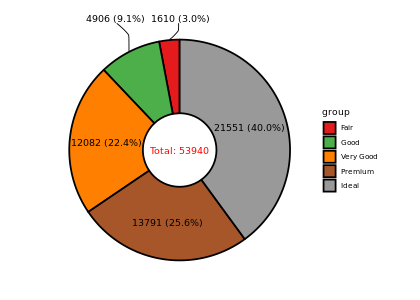``````ggdonut(data = diamonds, group_key = "cut", count_type = "full",
label_info = "all", label_type = "horizon",
label_size = 4, label_pos = "in", label_threshold = 10)``````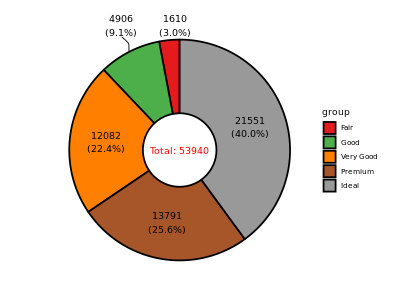### 3D pie plot

``````p1= ggpie3D(data = diamonds, group_key = "cut", count_type = "full", tilt_degrees = -10, label_size=2) +
ggtitle("tilt_degrees = -10") +
theme(plot.title = element_text(hjust = 0.5))

p2= ggpie3D(data = diamonds, group_key = "cut", count_type = "full", tilt_degrees = -40, label_size=2) +
ggtitle("tilt_degrees = -40") +
theme(plot.title = element_text(hjust = 0.5))

p3= ggpie3D(data = diamonds, group_key = "cut", count_type = "full", tilt_degrees = -10,
start_degrees = 60, label_size=2) +
ggtitle("start_degrees = 60") +
theme(plot.title = element_text(hjust = 0.5))

p4= ggpie3D(data = diamonds, group_key = "cut", count_type = "full", tilt_degrees = -10,
start_degrees = 180, label_size=2) +
ggtitle("start_degrees = 180") +
theme(plot.title = element_text(hjust = 0.5))

cowplot::plot_grid(p1,p2,p3,p4,ncol = 2)``````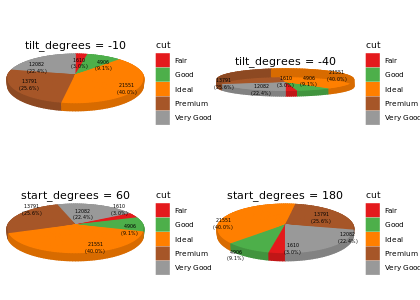### Nested pie/donut plot

It is often the case that we have two categorical variables and then need to plot for both of them, `ggnestedpie` is designed to deal with this.

#### inner circle and outer circle

inner circle label and no split, outer circle label and in pie plot

``````ggnestedpie(data = diamonds, group_key = c("cut", "color"), count_type = "full",
inner_label_info = "all", inner_label_split = NULL,inner_label_size = 2,
outer_label_type = "circle", outer_label_pos = "in", outer_label_info = "all")
#> Coordinate system already present. Adding new coordinate system, which will replace the existing one.``````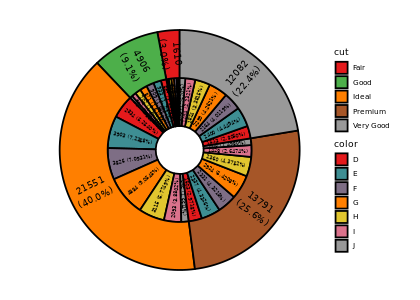inner circle label and no split, outer circle label and in pie plot, remove fraction below 1 of inner pie:

``````ggnestedpie(data = diamonds, group_key = c("cut", "color"), count_type = "full",
inner_label_info = "all", inner_label_split = NULL,
inner_label_threshold = 5, inner_label_size = 2,
outer_label_type = "circle", outer_label_pos = "in", outer_label_info = "all")
#> Coordinate system already present. Adding new coordinate system, which will replace the existing one.``````